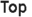# Growth

Cosma Home > Communication > Knowledge > Form > General > Foundation > Theory > System > Growth

# Spotlight

Wheat and chessboard problem

Pages

# Resources

These are organized by a classification scheme developed exclusively for Cosma. More…

## General

### Dictionary

growth : progressive development — Webster

### Encyclopedia

Growth refers to an increase in some quantity over time. The quantity can be:

Physical (e.g., growth in height, growth in an amount of money)
Abstract (e.g., a system becoming more complex, an organism becoming more mature).

It can also refer to the mode of growth, i.e. numeric models for describing how much a particular quantity grows over time. — Wikipedia

### Theory

Exponential growth is exhibited when the rate of change—the change per instant or unit of time—of the value of a mathematical function is proportional to the function’s current value, resulting in its value at any time being an exponential function of time, i.e., a function in which the time value is the exponent. Exponential decay occurs in the same way when the growth rate is negative. In the case of a discrete domain of definition with equal intervals, it is also called geometric growth or geometric decay, the function values forming a geometric progression. In either exponential growth or exponential decay, the ratio of the rate of change of the quantity to its current size remains constant over time. — Wikipedia

Exponential Growth and Decay (Math is Fun)

Quotations Page

## Participation

### Course

OER Commons: Open Educational Resources

ISBNdb

### Document

USA.gov